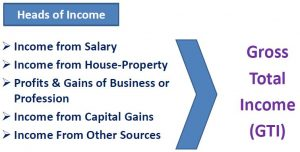# Difference between Gross Total Income and Total Income, Gross Total Income Vs Total IncomeGross Total Income (GTI) : Gross Total Income or GTI is the aggregate income earned from all heads of income, after giving effect of  clubbing of income and set-off or carry forward of losses.

There are 5 heads of Income :

(i) Salary , (ii) House Property (Rental Income), (iii) Business and Profession, (iv) Capital Gains, (v) Income from Other SourcesTotal Income (TI) : Total Income or TI is the income of an assessee on which TAX is calculated. It is the income after substracting DEDUCTIONS from Gross Total Income.

 Gross Total Income Less : Deductions under chapter VI-A of Income Tax (i.e. deduction under Sec 80C to 80U) Total Income

Lets understand with a Comparison Chart :

 Basis of Comparison Gross Total Income Total Income Meaning Gross Total Income is the aggregate income of a person, arrived after adding up income from all the five sources. Total Income refers to that income of the assessee on which the tax liability is calculated. Deductions Income before making deductions under Chapter VI-A Income after making deductions under Chapter VI-A Tax Tax is not levied on this income. Tax is levied on this income.